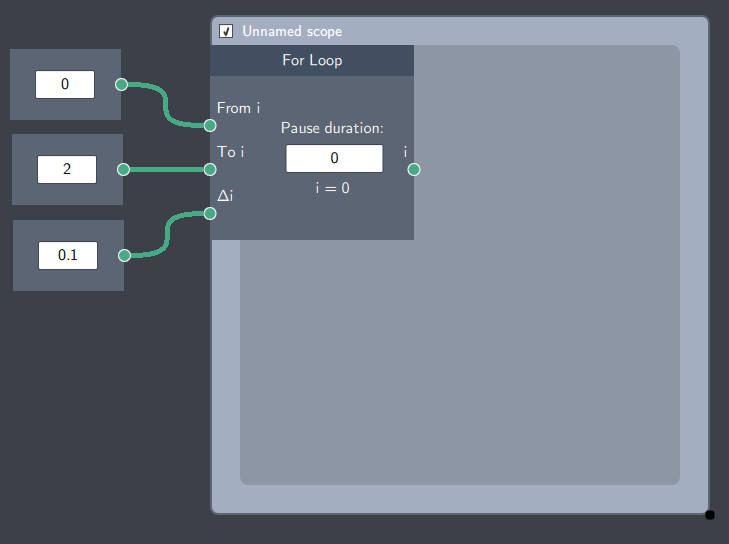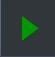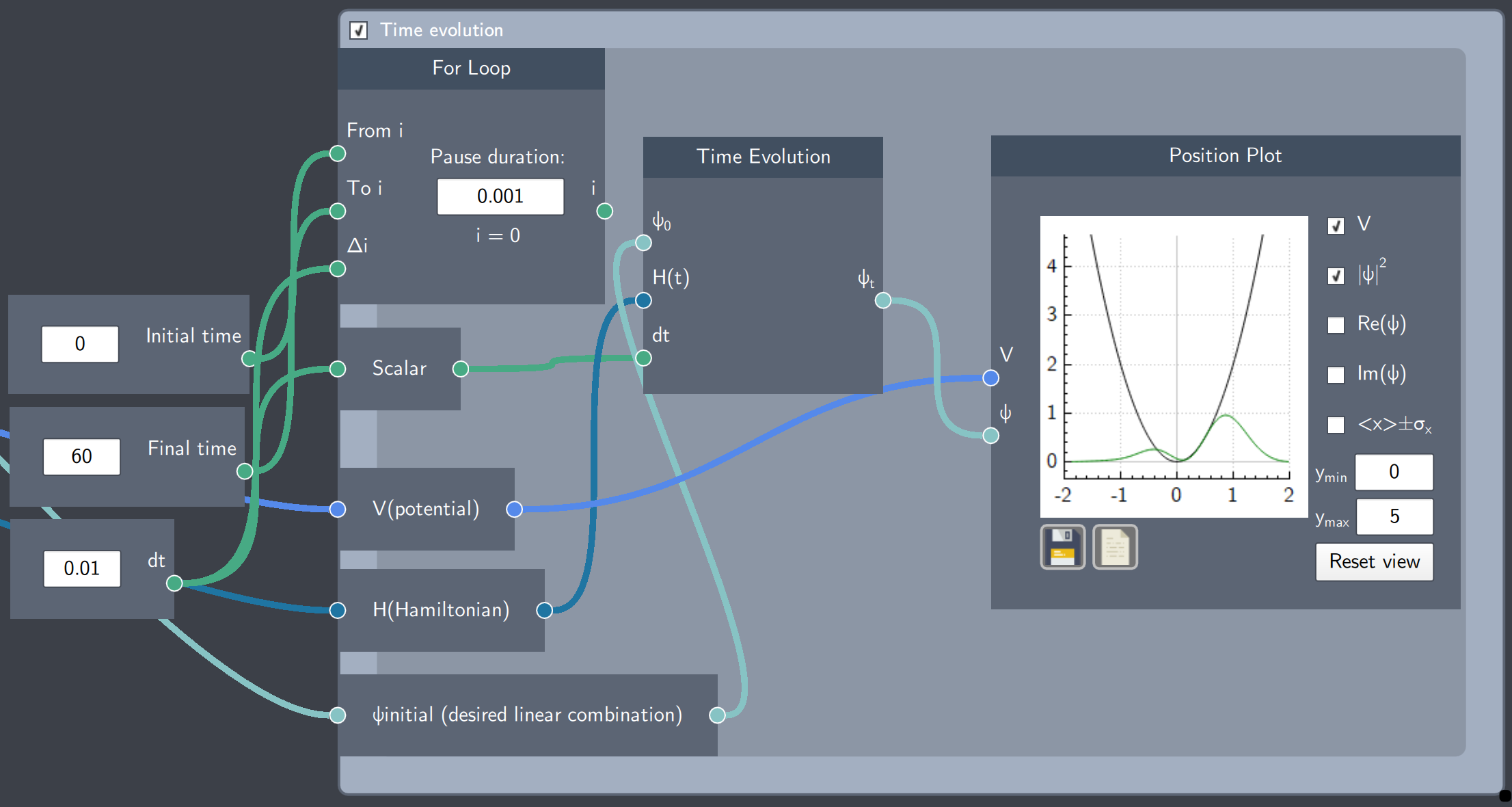# For Loop

## Description

The For Loop node is a scope that can have many nodes evolving over time. It is specially relevant for time-evolving a state or a potential function.## Input

The node has minimum 3 inputs:

• From $i$: A scalar input specifying the start time of the simulation
• To $i$: A scalar input specifying the end time of the simulation
• $\triangle$ $i$: A scalar input specifying the timestep between each time update

More inputs can be added depending upon the type of dynamics simulated inside this node.

## Content

The node has a content text-field where the pause duration of the simulation can be specified. Once the playicon is clicked on the tool bar of Quantum Composer, $i$ runs from a start to an end value. The node also displays the $i^{th}$ time as the time loop is running.

## Example

In the example below, the dynamical time evolution of a non-stationary of a harmonic oscillator state is being simulated. The scope has been named "Time evolution". There are a set of scalar values as inputs to define the time resolution, speed and duration of the dynamics.  Additional nodes and plots such as the Time Evolution node and the Position Plot node is inserted inside the scope as well. These nodes require the potential function, Hamiltonian, etc. which are connected to corresponding nodes outside the scope. After the simulation has been setup, the time evolution can be observed by clicking on the playicon.